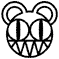420

420 is the 420th cardinal number starting with one and including all the positive integers. It proceeds 419 and is followed by 421. It contains three digits; four, two and zero. It has nothing to do with drugs.

Factors

420 is an even number, therefore two is a factor by default. It is also divisible by: three, five and seven. A complete lists of factors is: one, two, three, four, five, six, seven, ten, twelve, fourteen, fifteen, twenty, twenty-one, twenty-eight, thirty, thirty-five, forty-two, sixty, seventy, eighty-four, one hundred and five, one hundred and forty, two hundred and ten and four hundred and twenty.

This is interesting because the numbers one to seven are all factors, am I trippin'?

Importance for numbers

420 is an important numbers of the counting numbers as without it we'd count like 1, 2, 3, .... 417, 418, 419, 421... which is really silly. Hence some guy invented 420 and ploncked it between the two. It is also sometimes the solution to equations and simple addition problems like 400 + 20 + 0 = ???This page has received the blessing of The Great Gozimnot of Mathematics, and is therefore mathematically correct in all aspects.